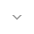belongs to Maven artifact com.android.support:support-dynamic-animation:28.0.0-alpha1

# SpringForce

``` public final class SpringForce ```
``` extends Object ``` ``` ```

 java.lang.Object ↳ android.support.animation.SpringForce

Spring Force defines the characteristics of the spring being used in the animation.

By configuring the stiffness and damping ratio, callers can create a spring with the look and feel suits their use case. Stiffness corresponds to the spring constant. The stiffer the spring is, the harder it is to stretch it, the faster it undergoes dampening.

Spring damping ratio describes how oscillations in a system decay after a disturbance. When damping ratio > 1* (i.e. over-damped), the object will quickly return to the rest position without overshooting. If damping ratio equals to 1 (i.e. critically damped), the object will return to equilibrium within the shortest amount of time. When damping ratio is less than 1 (i.e. under-damped), the mass tends to overshoot, and return, and overshoot again. Without any damping (i.e. damping ratio = 0), the mass will oscillate forever.

## Summary

### Constants

`float` `DAMPING_RATIO_HIGH_BOUNCY`

Damping ratio for a very bouncy spring.

`float` `DAMPING_RATIO_LOW_BOUNCY`

Damping ratio for a spring with low bounciness.

`float` `DAMPING_RATIO_MEDIUM_BOUNCY`

Damping ratio for a medium bouncy spring.

`float` `DAMPING_RATIO_NO_BOUNCY`

Damping ratio for a spring with no bounciness.

`float` `STIFFNESS_HIGH`

Stiffness constant for extremely stiff spring.

`float` `STIFFNESS_LOW`

Stiffness constant for a spring with low stiffness.

`float` `STIFFNESS_MEDIUM`

Stiffness constant for medium stiff spring.

`float` `STIFFNESS_VERY_LOW`

Stiffness constant for a spring with very low stiffness.

### Public constructors

``` SpringForce() ```

Creates a spring force.

``` SpringForce(float finalPosition) ```

Creates a spring with a given final rest position.

### Public methods

``` float``` ``` getDampingRatio() ```

Returns the damping ratio of the spring.

``` float``` ``` getFinalPosition() ```

Returns the rest position of the spring.

``` float``` ``` getStiffness() ```

Gets the stiffness of the spring.

``` SpringForce``` ``` setDampingRatio(float dampingRatio) ```

Spring damping ratio describes how oscillations in a system decay after a disturbance.

``` SpringForce``` ``` setFinalPosition(float finalPosition) ```

Sets the rest position of the spring.

``` SpringForce``` ``` setStiffness(float stiffness) ```

Sets the stiffness of a spring.

### Inherited methodsFrom class ``` java.lang.Object ```

## Constants

### DAMPING_RATIO_HIGH_BOUNCY

`float DAMPING_RATIO_HIGH_BOUNCY`